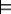### 12.4.1 Bottom-up Procedure with Variables

The propositional bottom-up proof procedure can be extended to Datalog by using ground instances of the clauses. A ground instance of a clause is obtained by uniformly substituting constants for the variables in the clause. The constants required are those appearing in the knowledge base or in the query. If there are no constants in the knowledge base or the query, one must be invented.

Example 12.18: Suppose the knowledge base is
q(a).
q(b).
r(a).
s(W) ←r(W).
p(X,Y) ←q(X) ∧s(Y).

The set of all ground instances is

q(a).
q(b).
r(a).
s(a) ←r(a).
s(b) ←r(b).
p(a,a) ←q(a) ∧s(a).
p(a,b) ←q(a) ∧s(b).
p(b,a) ←q(b) ∧s(a).
p(b,b) ←q(b) ∧s(b).

The propositional bottom-up proof procedure of Section 5.2.2.1 can be applied to the grounding to derive q(a), q(b), r(a), s(a), p(a,a), and p(b,a) as the ground instances that are logical consequences.

Example 12.19: Suppose the knowledge base is
p(X,Y).
g ←p(W,W).

The bottom-up proof procedure must invent a new constant symbol, say c. The set of all ground instances is then

p(c,c).
g ←p(c,c).

The propositional bottom-up proof procedure will derive p(c,c) and g.

If the query was ask p(a,d), the set of ground instances would change to reflect these constants.

The bottom-up proof procedure applied to the grounding of the knowledge base is sound, because each instance of each rule is true in every model. This procedure is essentially the same as the variable-free case, but it uses the set of ground instances of the clauses, all of which are true by definition.

This procedure is also complete for ground atoms. That is, if a ground atom is a consequence of the knowledge base, it will eventually be derived. To prove this, as in the propositional case, we construct a particular generic model. A model must specify what the constants denote. A Herbrand interpretation is an interpretation where the the domain is symbolic and consists of all constants of the language. An individual is invented if there are no constants. In a Herbrand interpretation, each constant denotes itself.

Consider the Herbrand interpretation where the ground instances of the relations that are eventually derived by the bottom-up procedure with a fair selection rule are true. It is easy to see that this Herbrand interpretation is a model of the rules given. As in the variable-free case, it is a minimal model in that it has the fewest true atoms of any model. If KBg for ground atom g, then g is true in the minimal model and, thus, is eventually derived.

Example 12.20: Consider the clauses of Figure 12.2. The bottom-up proof procedure can immediately derive each instance of imm_west given as a fact. Then you can add the imm_east clauses:
imm_east(r103,r101)
imm_east(r105,r103)
imm_east(r107,r105)
imm_east(r109,r107)
imm_east(r111,r109)
imm_east(r129,r131)
imm_east(r127,r129)
imm_east(r125,r127)

Next, the next_door relations that follow can be added to the set of consequences, including

next_door(r101,r103)
next_door(r103,r101)

The two_door_east relations can be added to the set of consequences, including

two_door_east(r105,r101)
two_door_east(r107,r103)

Finally, the west relations that follow can be added to the set of consequences.